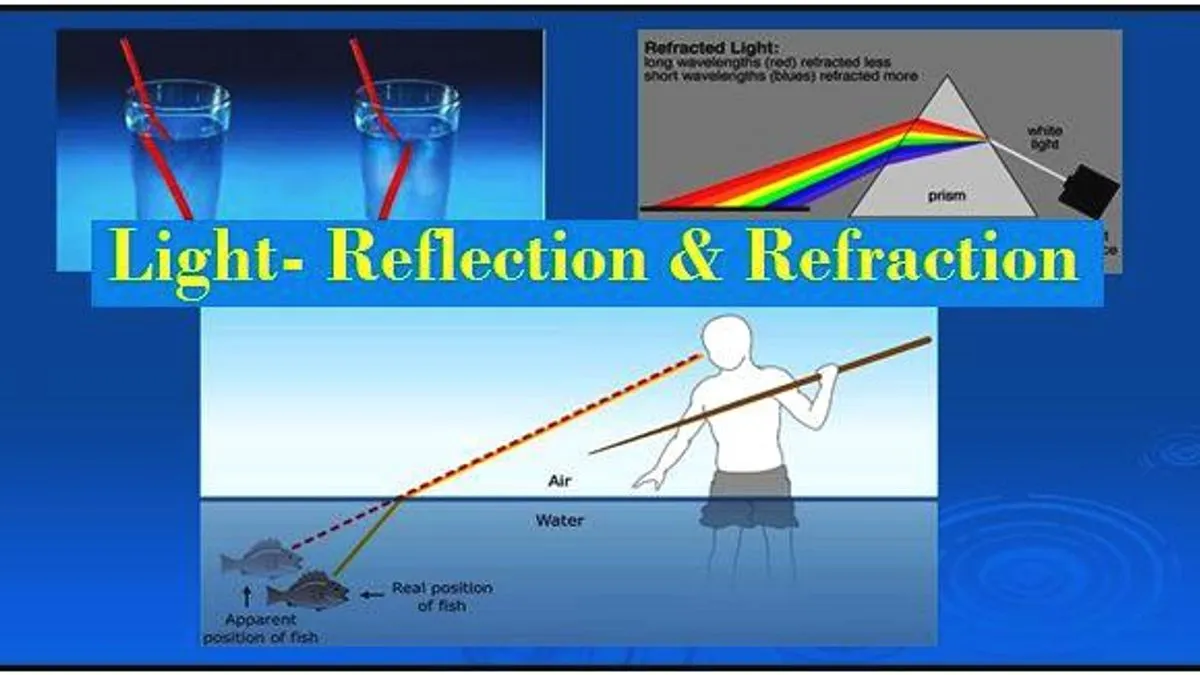# NCERT Solutions for Class 10 Science Chapter 9 Light- Reflection and Refraction, Download in PDF

## NCERT Solutions for Light- Reflection and Refraction: Get accurate NCERT Solutions for Class 10 Science Chapter 9 here to download in PDF. All the solutions are explained by subject experts and are best to clear concepts.NCERT Solutions for Class 10 Science Chapter Light- Reflection and Refraction

NCERT Solutions for Class 10 Light- Reflection and Refraction: To help students retrieve the right solutions for all the questions given in Class 10 Science NCERT book we have collated detailed and accurate answers here at jagranjosh.com. Prepared by subject matter experts, all the solutions will help students learn the right technique to write perfect answers in the board exams to obtain high score.

In this article we are providing the NCERT solutions for Class 10 Science Chapter 9: Light- Reflection and Refraction. Our subject experts have reviewed these NCERT solutions to provide error-free content which will make it easy for you to prepare easily and effectively for the class 10 board exams. All the class 10 Science NCERT solutions have been collated in PDF format which students may easily download for free.

Check NCERT Solutions for Class 10 Science Chapter  Light- Reflection and Refraction below:

Question 1: Define the principal focus of a concave mirror.

Solution: A point on principal axis on which parallel light rays converges after reflecting from the mirror is called the principal focus of a concave mirror.

Question 2: The radius of curvature of a spherical mirror is 20 cm. What is its focal length?

Solution:

Radius of curvature, R = 20 cm

Radius of curvature of a spherical mirror = 2 × Focal length (f)

R = 2f
⇒ f = R/2
⇒ f = 20/2 = 10 cm

Hence, the focal length of the given spherical mirror is 10 cm.

Question 3: Name a mirror that can give an erect and enlarged image of an object.

Solution: When an object is placed between the pole and the principal focus of a concave mirror, virtual, erect, and enlarged image is formed.

Question 4: Why do we prefer a convex mirror as a rear-view mirror in vehicles?

Solution: Convex mirror forms a virtual, erect, and diminished image of the objects that are placed in front of it. These mirrors give a wide field of view, which allows the driver to see most of the traffic behind him. Thus, they are preferred as a rear-view mirror in vehicles.

Question 5: Find the focal length of a convex mirror whose radius of curvature is 32 cm.

Solution:

Since the image formed here is real so it is inverted.

Therefore, Magnification produced, m = –3

Object distance, u = –10cm

Image distance, v = ?

Now, magnification produced by a spherical mirror is:

m = −Image distance/Object distance

⇒ m = −v/u

⇒ −3 = −v/−10

⇒  v = −30cm

Get the complete the NCERT solutions for Class 10: Light- Reflection and Refraction, from the following link:

Before solving the questions given in the Class 10 Science NCERT chapter- Light- Reflection and Refraction, students should thoroughly read this chapter to grasp all the concepts explained in it. This will help you understand the problem discussed in a question and then come up with an appropriate answer.

Main topics discussed in Class 10 Science chapter: Light- Reflection and Refraction are:

• Reflection of light
• Spherical mirrors
• Image formation by spherical mirrors
• Sign convention for spherical mirrors
• Mirror formula and magnification
• Refraction of light
• Refraction through a rectangular glass slab
• Refractive index
• Refraction by spherical lenses
• Image formation by lenses and their ray diagrams
• Sign convention for spherical lenses
• Lens formula and magnification
• Power of a lens

Check NCERT Books and Solutions from Class 4 to Class 12

We at Jagran Josh, provide the latest version of NCERT Books and NCERT solutions for all major subjects in class 4 to class 12. All the solutions have been prepared by the subject experts and are provided with detailed and appropriate explanation. Students must check these Free NCERT solutions to know the perfect answers for all questions given in NCERT books.

 Class 4 NCERT Book for Class 4 Maths NCERT Solutions for Class 4 Maths NCERT Book for Class 4 EVS NCERT Solutions for Class 4 EVS Class 5 NCERT Book for Class 5 Maths NCERT Solutions for Class 5 Maths NCERT Book for Class 5 EVS NCERT Solutions for Class 5 EVS Class 6 NCERT Book for class 6 Maths NCERT Solutions for Class 6 Maths NCERT Book for Class 6 Science NCERT Solutions for Class 6 Science Class 7 NCERT Book for Class 7 Science NCERT Solutions for Class 7 Science Class 8 NCERT Book for Class 8 Maths NCERT Solutions for Class 8 Maths NCERT Book for Class 8 Science NCERT Solutions for Class 8 Science Class 9 NCERT Book for class 9 Maths NCERT Solutions for Class 9 Maths NCERT Book for class 9 Science NCERT Solutions for Class 9 Science Class 10 NCERT Book for class 10 Maths NCERT Solutions for Class 10 Maths NCERT Book for class 10 Science NCERT Solutions for Class 10 Science NCERT Books and Solutions for Class 11 NCERT Books and Solutions for Class 12

Keep visiting Jagran Josh to get latest updates and reliable study material for preparations of all school and board examinations.

Get here latest School, CBSE and Government Jobs notification in English and Hindi for Sarkari Naukari and Sarkari Result. Download the Jagran Josh Sarkari Naukri App.

## Trending Categories

This website uses cookie or similar technologies, to enhance your browsing experience and provide personalised recommendations. By continuing to use our website, you agree to our Privacy Policy and Cookie Policy.